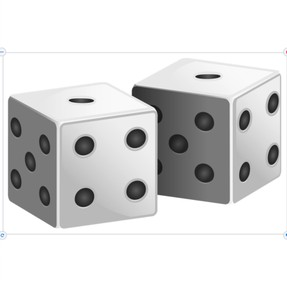Recognizing Dice to 12

# Recognizing Dice to 12

Recognizing dice to 12

No account needed.8,000 schools use Gynzy92,000 teachers use Gynzy1,600,000 students use Gynzy

## General

Students learn to recognize 2-12 on dice.

K.CC.B.4

## Relevance

It is useful to recognize a number at once on dice so you don't need to count all the dots. You need this, for example, to play a game with dice and quickly know how many spaces you can move.

## Introduction

The interactive whiteboard shows dice from 1 to 6. Refresh dice patterns and numbers 1-6, asking them to say or write the number of the dice as quickly as possible. Encourage students to recognize pattern rather than counting dots.

## Development

The interactive whiteboard shows images of dice with the amounts written under them. Refresh that numbers are linked to the patterns of dots on the dice. Encourage them to recognize pattern rather than count dots. Tell students to recognize amounts from both dice and then count them up together. Next practice recognizing amounts from 2-12 with dice. The interactive whiteboard has three sets of dice with three numbers, the students must determine which numbers match the dice and draw a line between pairs.

To check for understanding of dice to 12, you can ask the following questions:
Are the dots always in the same place?
Which number belongs to which dice?
How can you tell how many dots are on two dice?

## Guided Practice

Students determine which two dice belong to a number by choosing from two sets of dice. Next they see two dice, and need to determine the matching number. In the third exercise, students determine which dice add to 9 from 5 possible dice. Encourage the students to recognize amounts rather than count dots.

## Closing

Repeat the goal and the value of knowing this skill. Students will see three numbers with dice that can be dragged on the interactive whiteboard. They must determine which two dice are needed to create the given number. Encourage the students to make different combinations. The number 8 for example can be dice with 6 and 2 dots, 5 and 3 dots, but also 4 and 4 dots. Discuss the different possibilities. Students can practice recognizing 2-12 on the dice in game form. Have the students form pairs and roll dice, where the partner must name the total as quickly as possible.

## Teaching Tip

Students who have difficulty with recognizing 1-12 on dice can practice with dice to 6. You can use flash cards for this, with the number on one side and the dots on the dice on the other.

## Instruction materials

dice

### The online teaching platform for interactive whiteboards and displays in schools

• Save time building lessons

• Manage the classroom more efficiently

• Increase student engagement